Home » Math » FREE Step by Step Directions For Dividing by 10 Using Arrays

# FREE Step by Step Directions For Dividing by 10 Using Arrays

Grab these step by step directions for dividing by 10 using arrays. Children will learn how to use base ten blocks to create an array while dividing.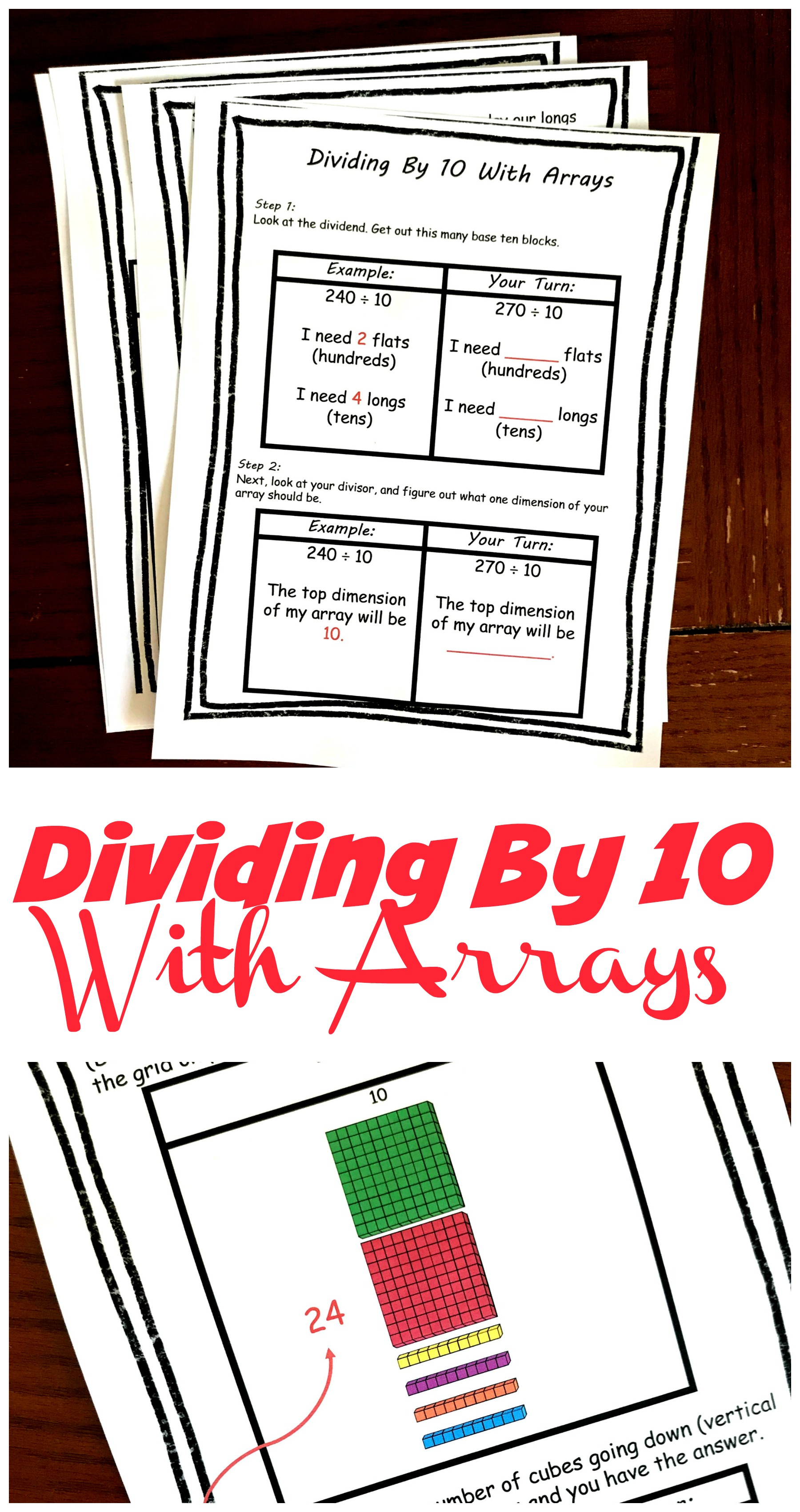It is incredibly important for students to understand what happens when you multiply and divide by 10. You see, it is the foundation of place value.

Let’s start with the digit 2 in the one’s place. I can show this by getting out two cubes. But let’s say I want to move the digit 2 to the tens place. How would I do that?

I would multiply 2 by 10. 2 x 10 = 20. When I move the digit 2 one place to the left I am multiplying by 10.

Now let’s say that the digit 5 is in the hundreds place. I can represent this digit by getting out 5 flats to show five hundred. So how do I move it one place to the right???

I divide by 10. 500 ÷ 10 = 50. The digit 5 in the tens place equal 50.

And today, we are going to explore dividing by 10 and helping children visualize the answers.

## Prep- Work

Very little prep-work is needed for this activity.

1. First, print off the pages.
2. Second, punch holes or provide glue so that children can put the papers in their math notebooks.
3. Finally, gather up colored pencils and base ten blocks……

And you are ready to go.

## Dividing by 10

Now it’s time to divide.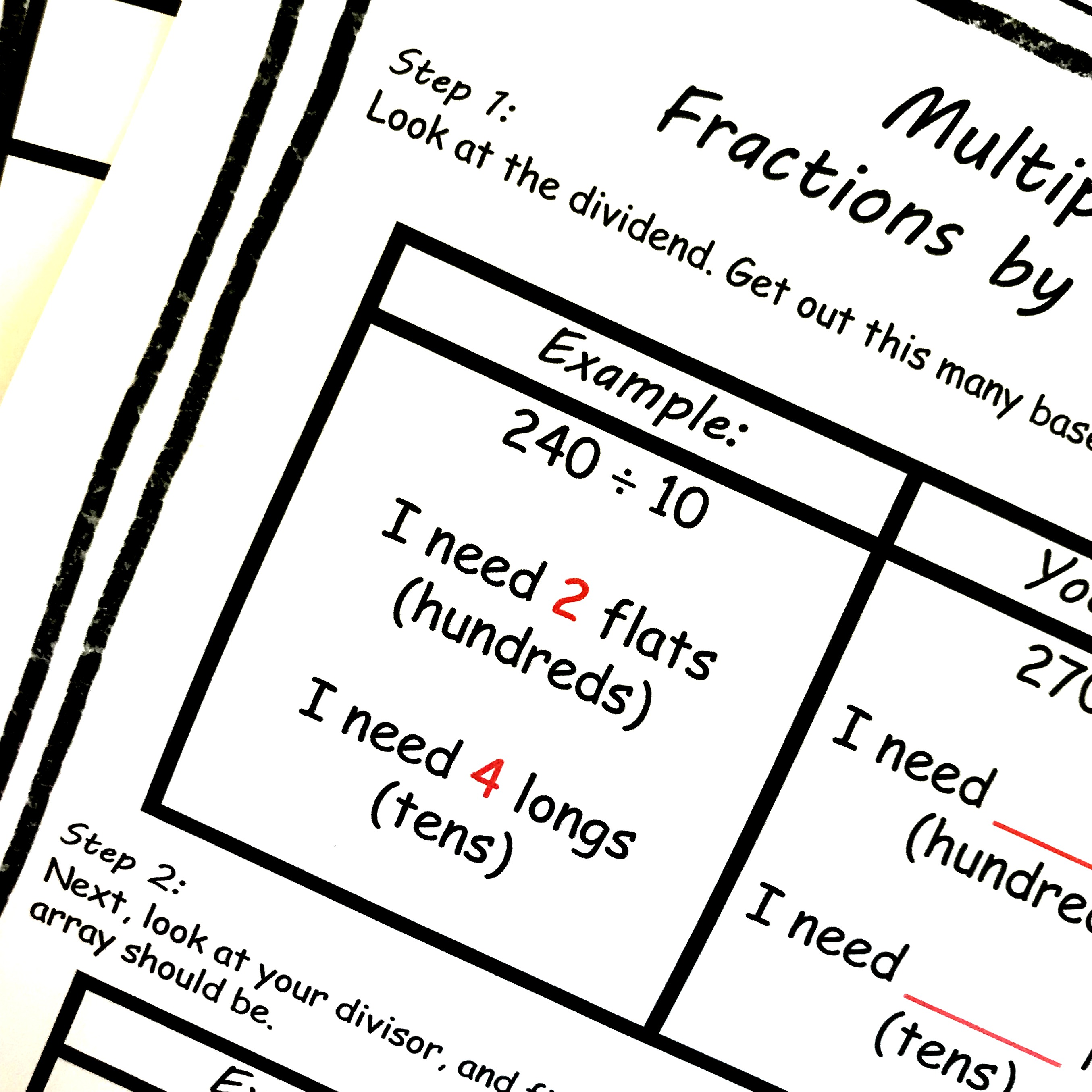We begin by looking at the dividend (the number being divided up) and getting out that many base ten blocks. In the example above, my dividend is 240 so I don’t need any cubes, I need four longs, and I need two flats.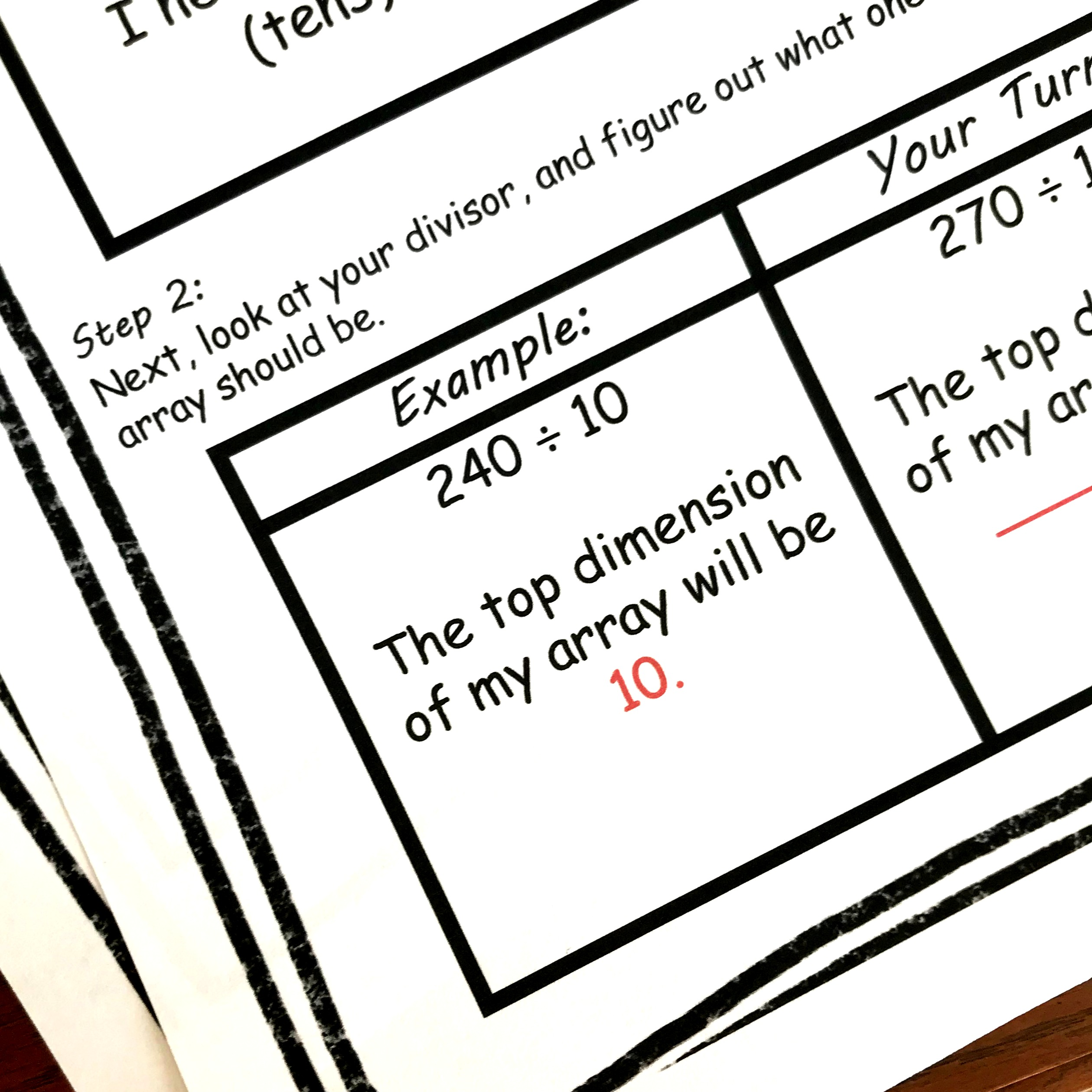Next, I look at my divisor (how many groups there will be) and figure out what the top dimension of my array will be. Since the divisor is 10, I know that my array will have 10 cubes going across the top.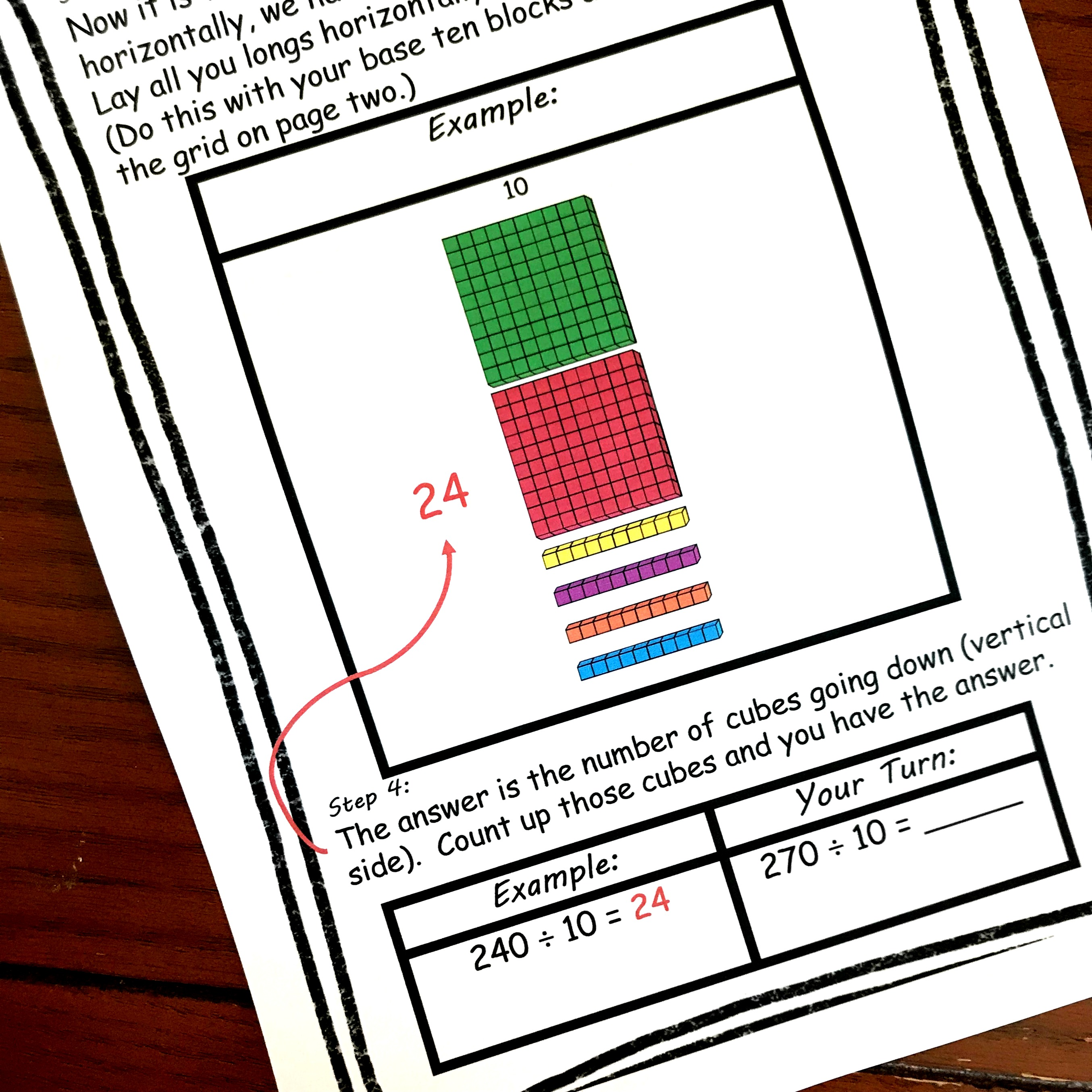Now it is time to build the array. A flat has 10 cubes across the top, so I simply line them up vertically down my page. Now I have two four longs. When they are turned horizontally those longs have 10 cubes and fit perfectly under the flats.

All that is left to do is figure out the dimensions of my array. I have 10 at the top, 24, down the side, and 240 cubes in the array. So……

10 x 24= 240

240/10 = 24

It is that simple to help children be able to visualize what is happening when they divide by 10.

You May Also Like:

Four other Activites to Practice Dividing By 10: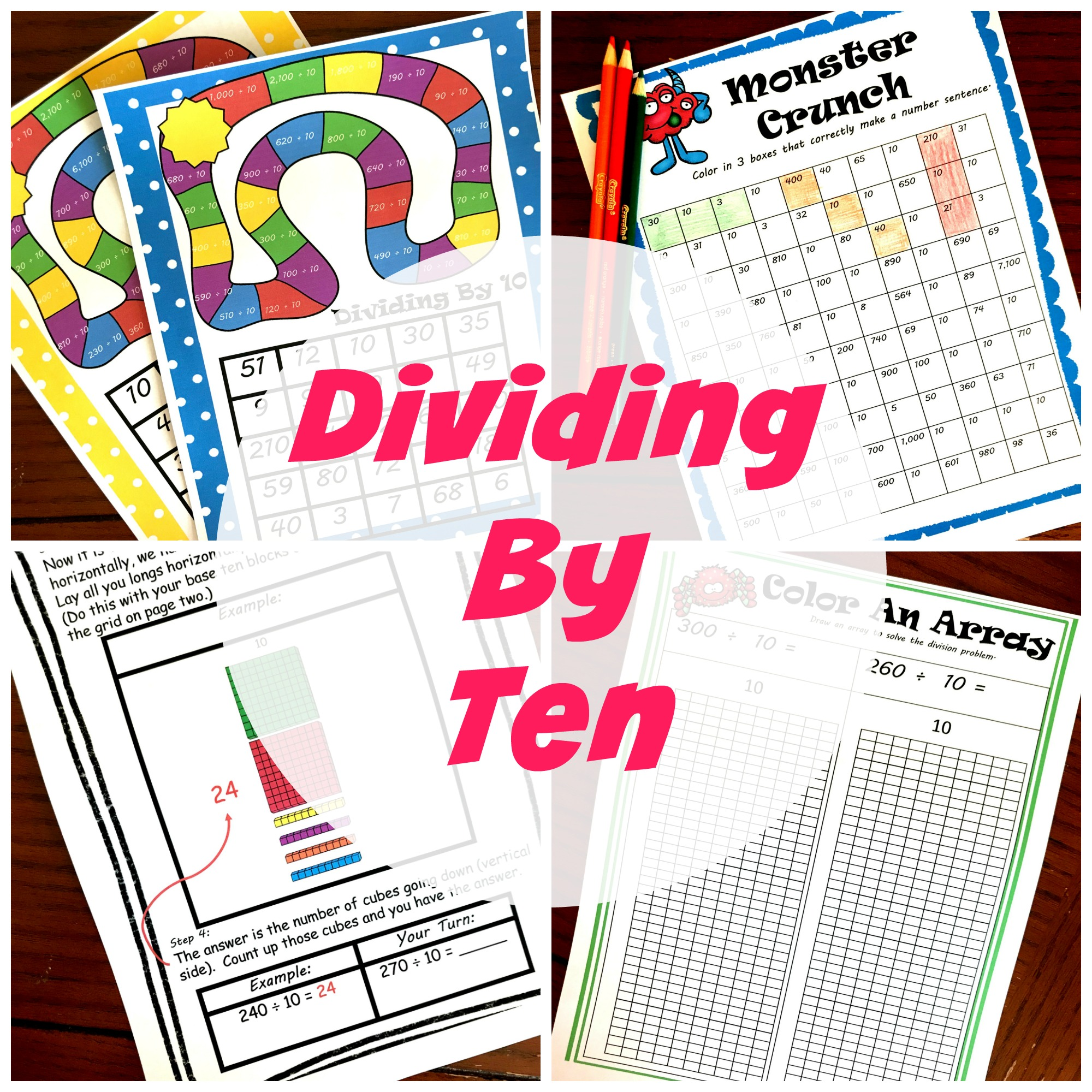Practice Multiplying By 10: